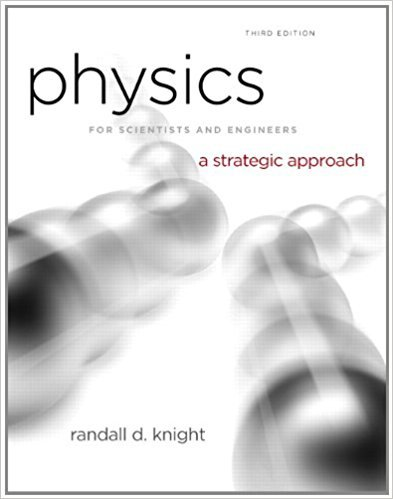×
Get Full Access to Physics For Scientists And Engineers: A Strategic Approach With Modern Physics - 3 Edition - Chapter 10 - Problem 37p
Get Full Access to Physics For Scientists And Engineers: A Strategic Approach With Modern Physics - 3 Edition - Chapter 10 - Problem 37p

×

# A very slippery ice cube slides in a vertical plane aroundISBN: 9780321740908 69

## Solution for problem 37P Chapter 10

Physics for Scientists and Engineers: A Strategic Approach with Modern Physics | 3rd Edition

• Textbook Solutions
• 2901 Step-by-step solutions solved by professors and subject experts
• Get 24/7 help from StudySoup virtual teaching assistantsPhysics for Scientists and Engineers: A Strategic Approach with Modern Physics | 3rd Edition

4 5 1 337 Reviews
10
5
Problem 37P

Problem 37P

A very slippery ice cube slides in a vertical plane around the inside of a smooth, 20-cm-diameter horizontal pipe. The ice cube’s speed at the bottom of the circle is 3.0 m/s.

a. What is the ice cube’s speed at the top?

b. Find an algebraic expression for the ice cube’s speed when it is at angle 9, where the angle is measured counterclockwise from the bottom of the circle. Your expression should give 3.0 m/s for θ = 0° and your answer to part a for θ = 180°.

Step-by-Step Solution:

Solution 37P

Step 1 of 5

An ice cube sliding in a vertical plane inside a smooth horizontal pipe. We need to find (a) The speed of the ice cube at the top. (b) To find the algebraic expression for the speed of ice cube, and also to find the value atin part (a).

Step 2 of 5

Step 3 of 5

##### ISBN: 9780321740908

Unlock Textbook Solution